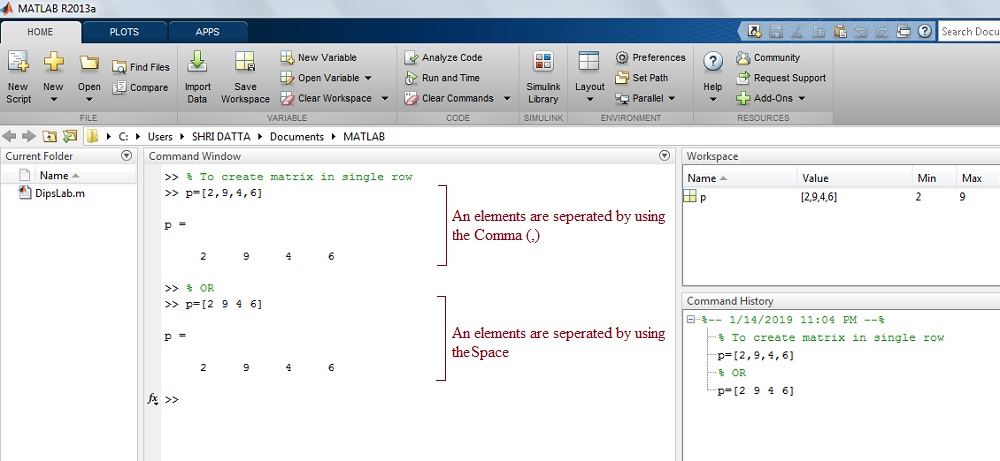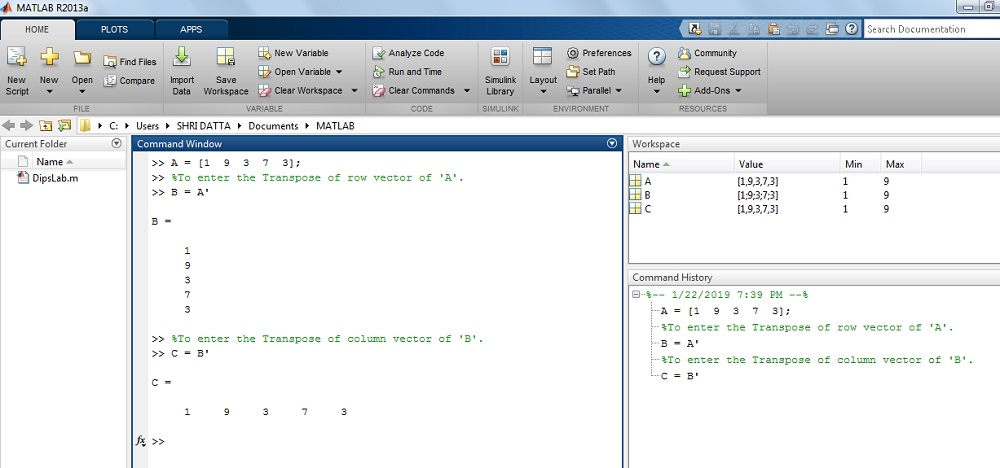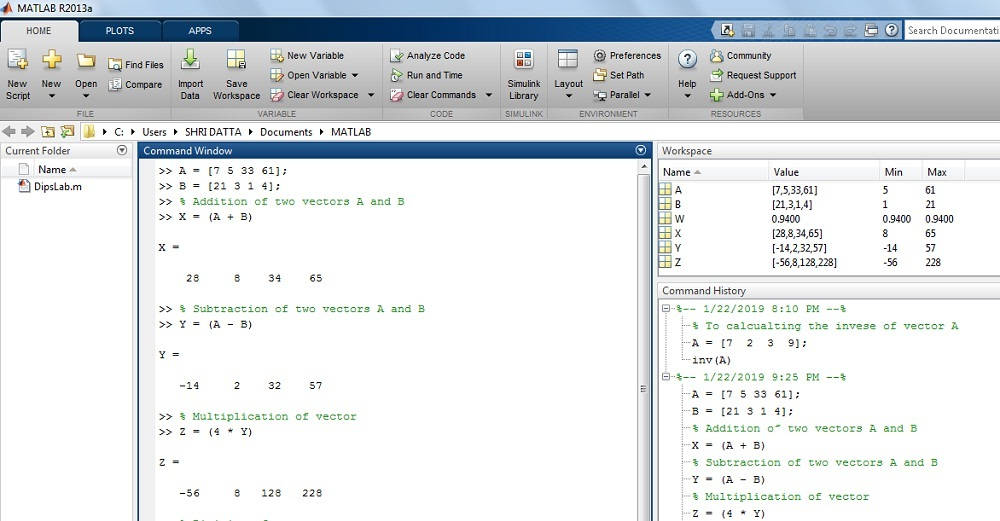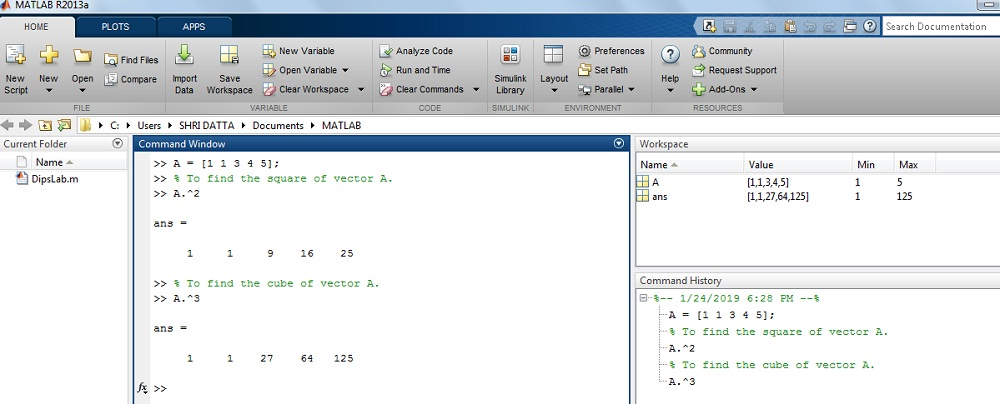# [Detail Guide] Create MATLAB Vector | Types | Mathematical OperationsThis latest tutorial is a part of the MATLAB vector where you will learn about generating and manipulating vectors along with multiple mathematical functions.

So here you go- ‘How to create a vector in MATLAB?’

So at the end of this tutorial, you will be able to create row and column vectors along with performing mathematical operations.

### INTRODUCTION

Vector is a special case of a matrix. It is a one-dimensional array consisting of the rows (m) and columns (n).

This term is also familiar with the vector in mathematics.

In the vector, the elements are separated by the commas or spaces or semicolon. It is enclosed by the square bracket ‘[ ]’.

Depending on the organization of the elements in the vectors, they are of two types.

### TYPES OF VECTOR

In MATLAB, the vector is classified into two types as given below.

• Row vector
• Column vector

Let us see, the step-by-step explanation of both vectors.

#### Row Vector:

In Row Vector, the set of all elements are placed in a horizontal position. These row elements are separated by the commas or line-space.

The general represent as,

```A = [x1, x2, x3, ....... xn] or [x1 x2 x3 ....... xn]

This is (1 × n) vector i.e. a single row consisting the n th term elements.```

The Generation of Row Vector:

Here, the row vector is saved as ‘p’.

The elements of the vector are separated by both forms like as commas and spaces.

```>>   p=[2, 9, 4, 6]

or

>>   p=[2 9 4 6]

p=

2   9   4   6```

Output in the MATLAB window:#### Column Vector:

In Column Vector, the set of all elements are shown in a vertical position. These column elements are separated by the semicolon (;).

The general represent as,

```A = [x1; x2; x3; ....... xm]

This is (m × 1) vector i.e. a single row consisting the m th term elements.```

The Generation of Column Vector:

Representing the column vector as a ‘q’ in MATLAB.

You can see, the elements of the column vector are separated by semicolumn (;).

```>> q = [1;  4;  7;  9]

q =

1

4

7

9```

Output in MATLAB Window:This is all about different types of MATLAB vectors.

Now we will see some mathematical operations on those MATLAB vectors.

#### TRANSPOSE OF VECTOR

The transpose of the vector is denoted by an apostrophe or single quote (‘) or power of T.

Transpose method is a mathematical operation where…

• the row vector is converted into the column vector or
• column vector converted into the row vector.

In general syntax form,

```A = [x1, x2, x3, ...... xn]'= [x1; x2; x3; ...... xn]

B = [x1; x2; x3; ...... xm]'= [x1, x2, x3, ...... xm]```

Example:

#### 1. How to convert row vector into the column vector?

You can use the transpose method to change the row vector into a column vector.

```>> A = [1  9  3  7  3]

>> B = A'

B =

1

9

3

7

3```

#### 2. How to convert a column vector into the row vector?

Same as above use transpose method to change the column vector into a row vector.

```>> C = B' C

= 1 9 3 7 3```

The result on the MATLAB window:Transpose is a simple and most common operation you can perform on MATLAB vectors.

Now, let us see, some other mathematical functions for arithmetic operation.

### MATHEMATICAL OPERATIONS ON VECTOR

In the last post, I have explained the multiple Mathematical functions and their syntax in MATLAB.

These mathematical functions and their syntax are useful in the vector operation as well.

#### 1. Arithmetic Operation of Vector:

The basic arithmetic operation consists of the addition (+), subtraction (-), multiplication (*), etc.

Here, I am doing some basic mathematical operations.

For Example:

```>> A = [7 5 33 61];

>> B =[21 3 1 4];

>> Addition of two vector A and B

>> X = (A + B)

X =
28 8 34 65

>> Substraction of two vector A and B

>> Y = (A-B)

Y =
-14 2 32 57

>> Mutliplication of vector

>> z=(4*y)

z =
-56 8 128 228```

The result on MATLAB display:#### 2. Length of Vector:

Length function is useful for calculating the number of elements in the vector.

In the MATLAB, there is a length method.

Example:

How to calculate the length of the vector in MATLAB?

Here is a syntax.

```>> X = [2 4 1 7 8];

>> length(X)

= 5```

The Result on MATLAB display:#### 3. Square and Cube (power) of the Vector:

In MATLAB, ‘.^’ (Dot power) is used for calculating the square and cube (or power) of the vector.

Example:

How to find the Square or Cube of the vector in MATLAB?

Here is a syntax.

```>> A = [1 1 3 4 5];

>> To find the square of vector A.

>> A.^2

= [1 1 9 16 25]

>> To find the cube of vector A.

>> A.^3

= [1 1 27 64 125]```

The Result on MATLAB display:#### 4. Square Root of the Vector:

Sqrt method is used for calculating the square root of the vector elements.

Example:

How to find the square root of the Vector in MATLAB?

Here is a syntax.

```>> X = [2, 4, 1, 7, 8];

>> sqrt(X)

= [1.4142 2.0000 1.0000 2.6458 2.8284]```

The Result on MATLAB display:#### 5. Minimum or Maximum element of the Vector:

min and max syntax use for calculating the minimum and maximum elements of the vector.

Example:

How to find the maximum and minimum element of the vector in MATLAB?

Here is a syntax.

```>> X = [2 4 1 7 8];

>> min(X)

= 1

>> max(X)

= 8```

The Result on MATLAB display:#### 6. Colon Function for Vector:

For MATLAB, the colon (:) function are express the sequence and position of the elements in the vector.

Example:

How to calculate the position and sequence of the element in the row vector?

Here is a syntax.

```>> X = [2 3 4 4  8 9 6];

>> To find the first and fifth postion of row element in a vector X.

>> X(1)

= 2

>> X(5)

=8

>> To find the first postion to fifth postion in vector.

>> X(1:5)

= [2 3 4 4 8]

>> To find the fifth posistion to last position in MATLAB.

>> X(4:end)

= [4 8 9 6]```

The result on MATLAB display:In this post, I covered all the basics of the vector in MATLAB and also explained some of the mathematic operations on MATLAB vector.

If you have any query related to this topic, let’s discuss this in the comment section below.

If You Appreciate What I Do Here On DipsLab, You Should Consider:

DipsLab is the fastest growing and most trusted community site for Electrical and Electronics Engineers. All the published articles are available FREELY to all.

If you like what you are reading, please consider buying me a coffee ( or 2 ) as a token of appreciation.

This will help me to continue my services and pay the bills.I’m thankful for your never-ending support.

### 4 thoughts on “[Detail Guide] Create MATLAB Vector | Types | Mathematical Operations”

1.I wanted to thank you for this fantastic read!
I absolutely loved every little bit of it.
I’ve got you book-marked to check out new stuff your post.

•You are most welcome, Stephen.

I started my Newsletter. Soon, I will send the updated electrical information and the tutorials in every week.

2.•